# Volume and Density Volume Capacity Volume is the

• Slides: 70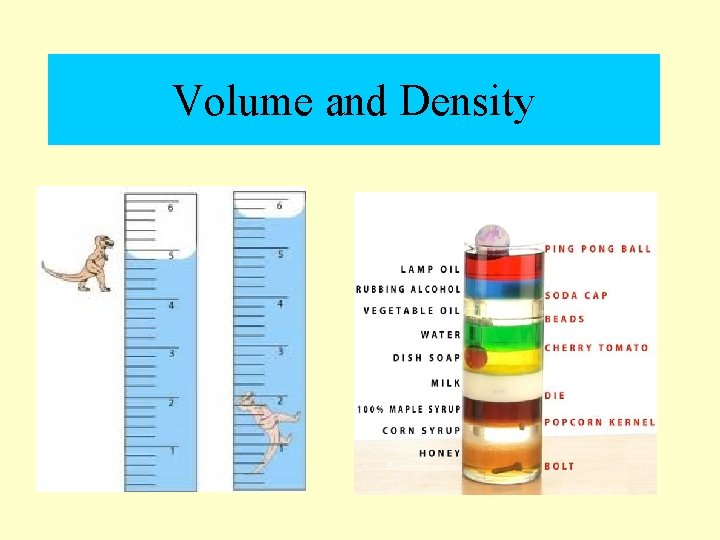Volume and Density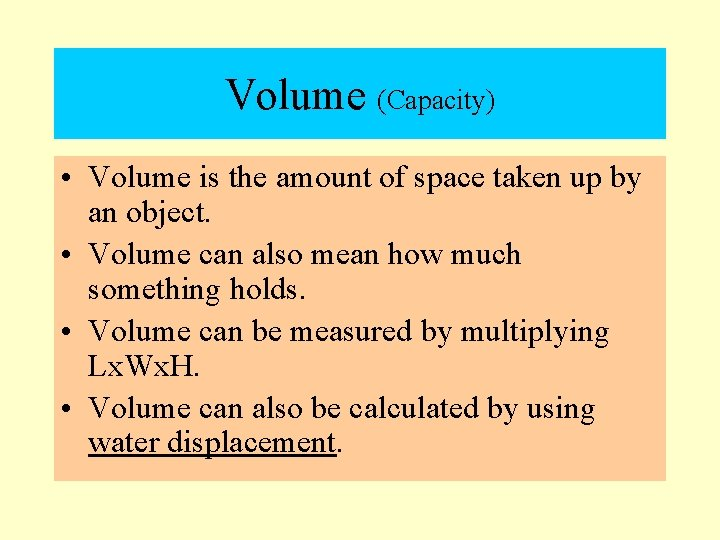Volume (Capacity) • Volume is the amount of space taken up by an object. • Volume can also mean how much something holds. • Volume can be measured by multiplying Lx. Wx. H. • Volume can also be calculated by using water displacement.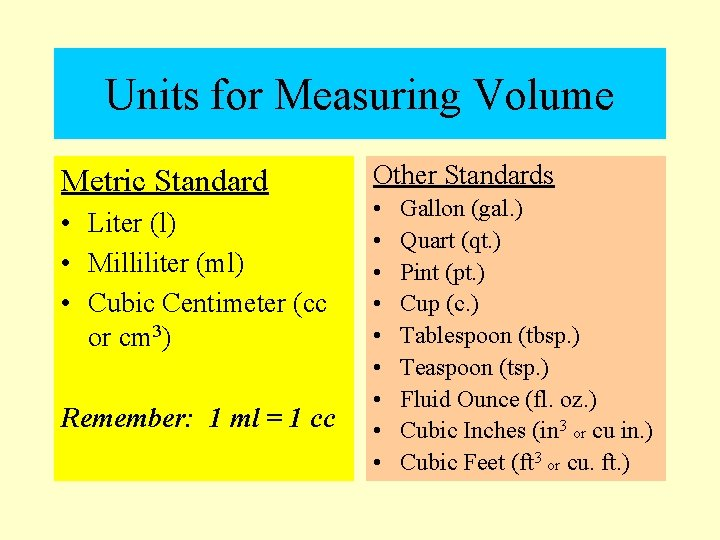Units for Measuring Volume Metric Standard • Liter (l) • Milliliter (ml) • Cubic Centimeter (cc or cm 3) Remember: 1 ml = 1 cc Other Standards • • • Gallon (gal. ) Quart (qt. ) Pint (pt. ) Cup (c. ) Tablespoon (tbsp. ) Teaspoon (tsp. ) Fluid Ounce (fl. oz. ) Cubic Inches (in 3 or cu in. ) Cubic Feet (ft 3 or cu. ft. )Using Water Displacement to Calculate Volume 1. Measure the level of the water in a container. 70 ml 60 ml 50 ml 40 ml 30 ml 20 ml 10 ml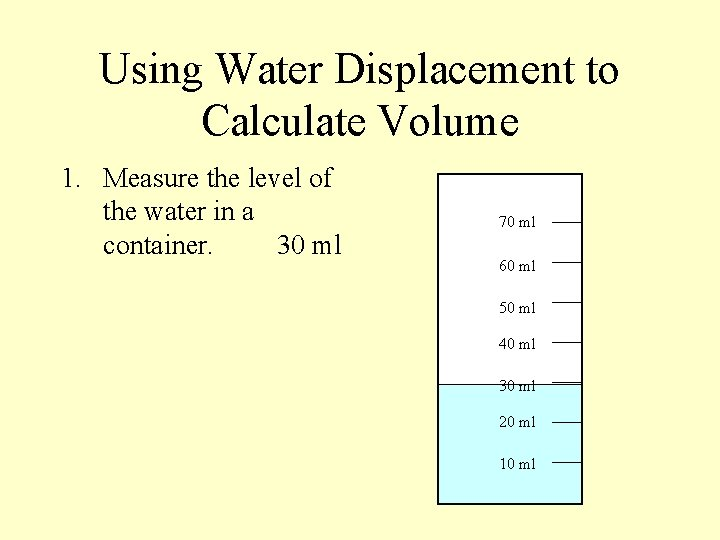Using Water Displacement to Calculate Volume 1. Measure the level of the water in a container. 30 ml 70 ml 60 ml 50 ml 40 ml 30 ml 20 ml 10 ml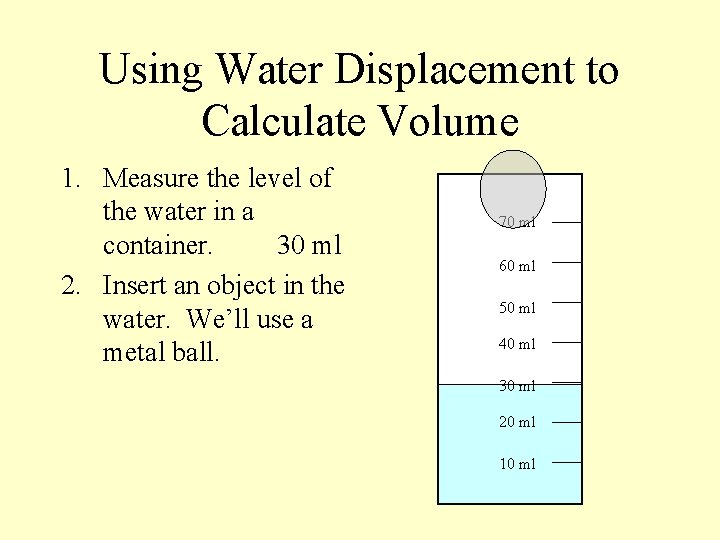Using Water Displacement to Calculate Volume 1. Measure the level of the water in a container. 30 ml 2. Insert an object in the water. We’ll use a metal ball. 70 ml 60 ml 50 ml 40 ml 30 ml 20 ml 10 mlUsing Water Displacement to Calculate Volume 1. Measure the level of the water in a container. 30 ml 2. Insert an object in the water. We’ll use a metal ball. 70 ml 60 ml 50 ml 40 ml 30 ml 20 ml 10 mlUsing Water Displacement to Calculate Volume 1. Measure the level of the water in a container. 30 ml 2. Insert an object in the water. We’ll use a metal ball. 70 ml 60 ml 50 ml 40 ml 30 ml 20 ml 10 mlUsing Water Displacement to Calculate Volume 1. Measure the level of the water in a container. 30 ml 2. Insert an object in the water. We’ll use a metal ball. 70 ml 60 ml 50 ml 40 ml 30 ml 20 ml 10 ml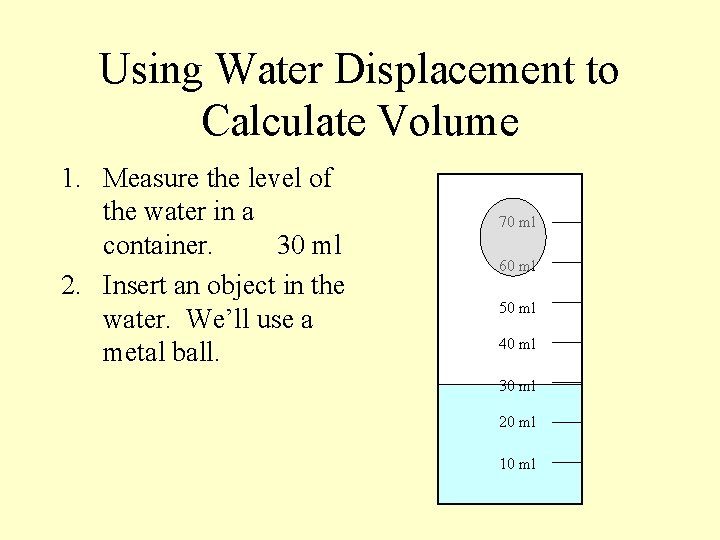Using Water Displacement to Calculate Volume 1. Measure the level of the water in a container. 30 ml 2. Insert an object in the water. We’ll use a metal ball. 70 ml 60 ml 50 ml 40 ml 30 ml 20 ml 10 ml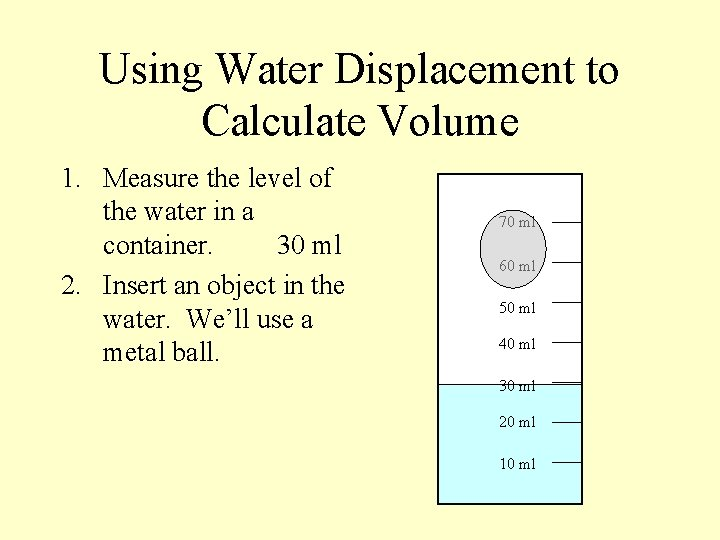Using Water Displacement to Calculate Volume 1. Measure the level of the water in a container. 30 ml 2. Insert an object in the water. We’ll use a metal ball. 70 ml 60 ml 50 ml 40 ml 30 ml 20 ml 10 mlUsing Water Displacement to Calculate Volume 1. Measure the level of the water in a container. 30 ml 2. Insert an object in the water. We’ll use a metal ball. 70 ml 60 ml 50 ml 40 ml 30 ml 20 ml 10 ml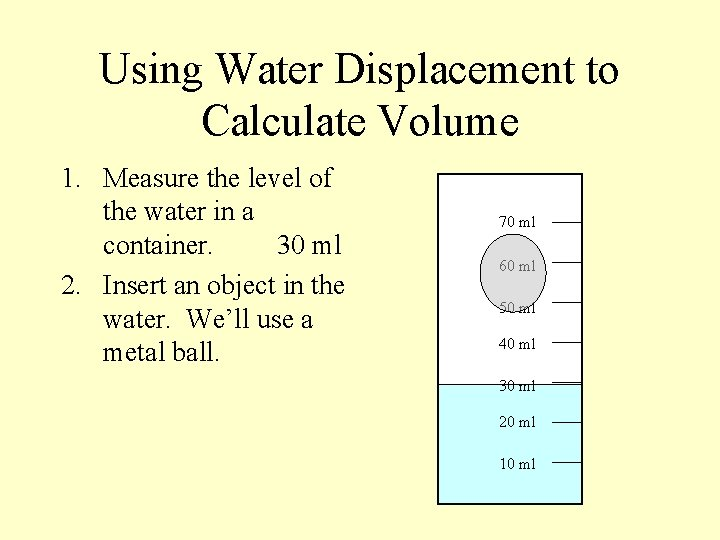Using Water Displacement to Calculate Volume 1. Measure the level of the water in a container. 30 ml 2. Insert an object in the water. We’ll use a metal ball. 70 ml 60 ml 50 ml 40 ml 30 ml 20 ml 10 ml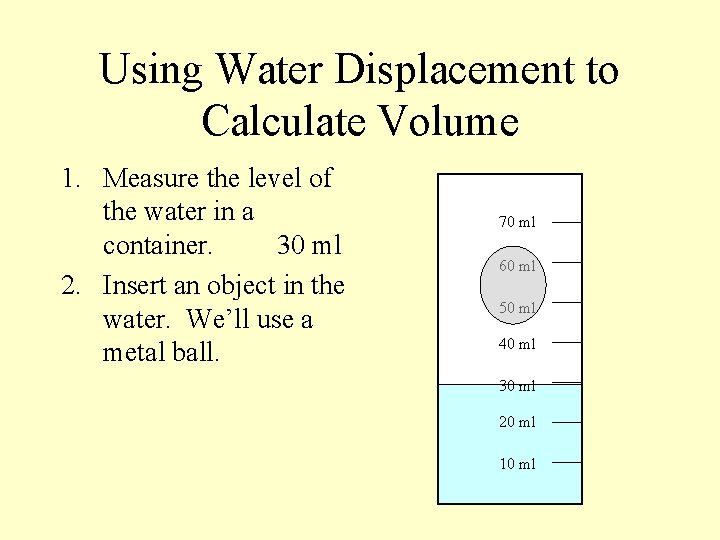Using Water Displacement to Calculate Volume 1. Measure the level of the water in a container. 30 ml 2. Insert an object in the water. We’ll use a metal ball. 70 ml 60 ml 50 ml 40 ml 30 ml 20 ml 10 ml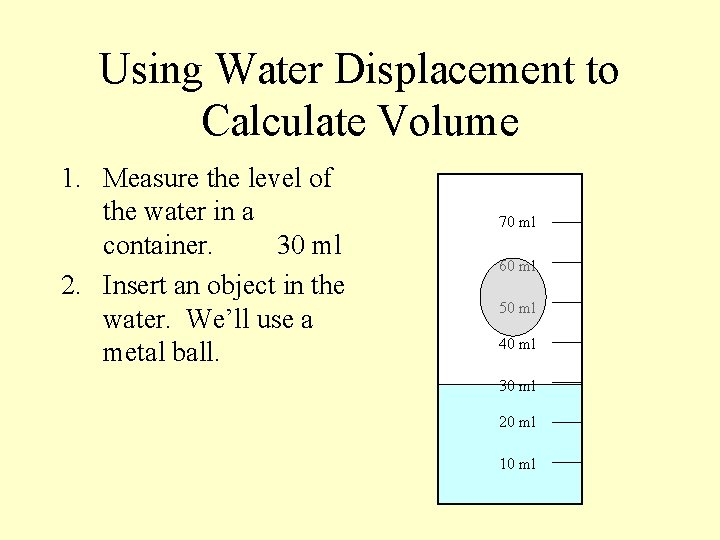Using Water Displacement to Calculate Volume 1. Measure the level of the water in a container. 30 ml 2. Insert an object in the water. We’ll use a metal ball. 70 ml 60 ml 50 ml 40 ml 30 ml 20 ml 10 ml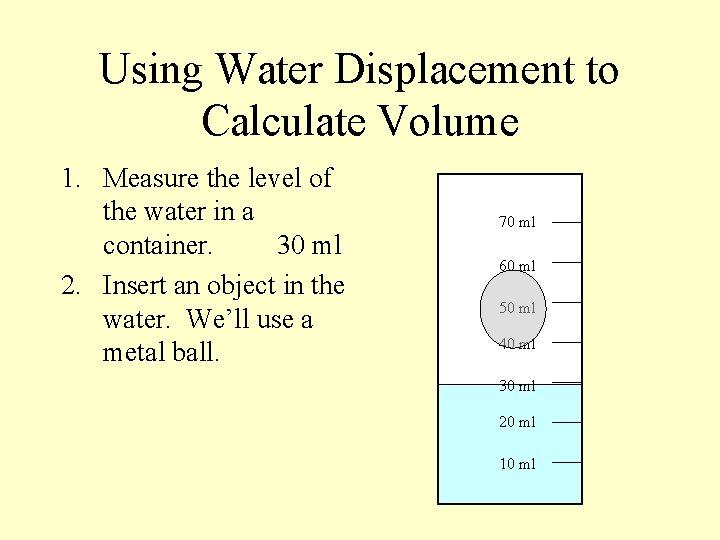Using Water Displacement to Calculate Volume 1. Measure the level of the water in a container. 30 ml 2. Insert an object in the water. We’ll use a metal ball. 70 ml 60 ml 50 ml 40 ml 30 ml 20 ml 10 ml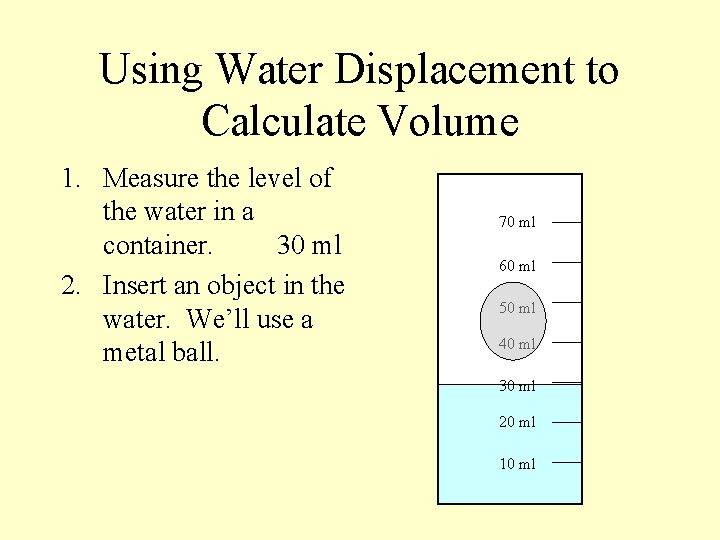Using Water Displacement to Calculate Volume 1. Measure the level of the water in a container. 30 ml 2. Insert an object in the water. We’ll use a metal ball. 70 ml 60 ml 50 ml 40 ml 30 ml 20 ml 10 ml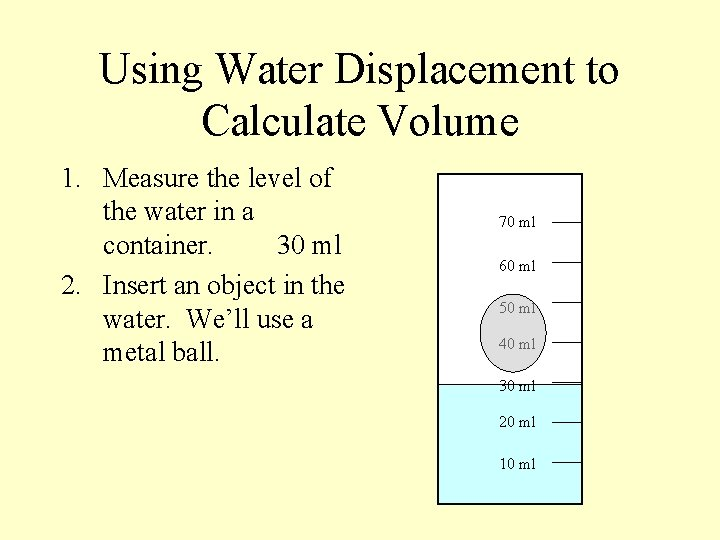Using Water Displacement to Calculate Volume 1. Measure the level of the water in a container. 30 ml 2. Insert an object in the water. We’ll use a metal ball. 70 ml 60 ml 50 ml 40 ml 30 ml 20 ml 10 mlUsing Water Displacement to Calculate Volume 1. Measure the level of the water in a container. 30 ml 2. Insert an object in the water. We’ll use a metal ball. 70 ml 60 ml 50 ml 40 ml 30 ml 20 ml 10 ml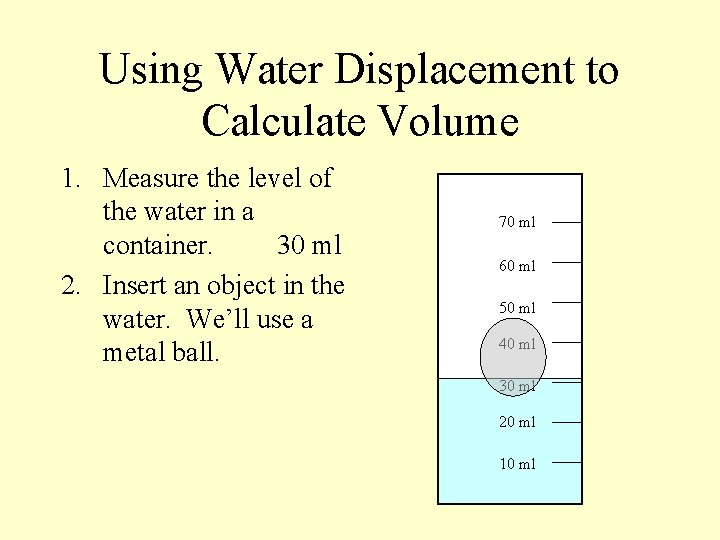Using Water Displacement to Calculate Volume 1. Measure the level of the water in a container. 30 ml 2. Insert an object in the water. We’ll use a metal ball. 70 ml 60 ml 50 ml 40 ml 30 ml 20 ml 10 mlUsing Water Displacement to Calculate Volume 1. Measure the level of the water in a container. 30 ml 2. Insert an object in the water. We’ll use a metal ball. 70 ml 60 ml 50 ml 40 ml 30 ml 20 ml 10 ml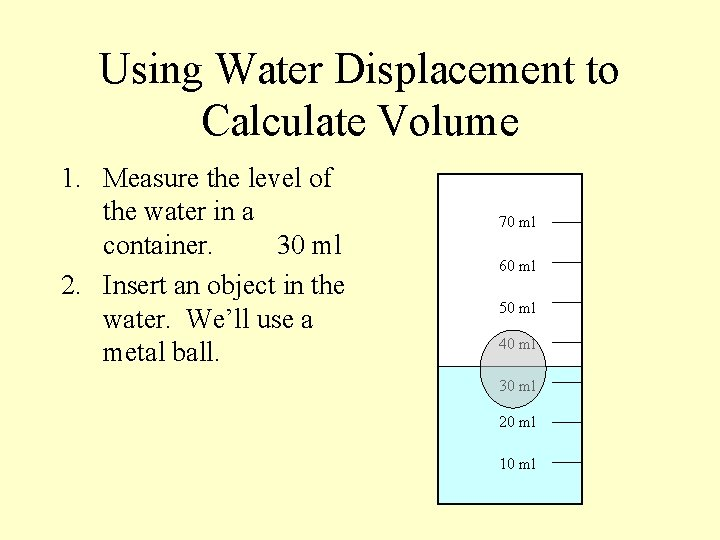Using Water Displacement to Calculate Volume 1. Measure the level of the water in a container. 30 ml 2. Insert an object in the water. We’ll use a metal ball. 70 ml 60 ml 50 ml 40 ml 30 ml 20 ml 10 ml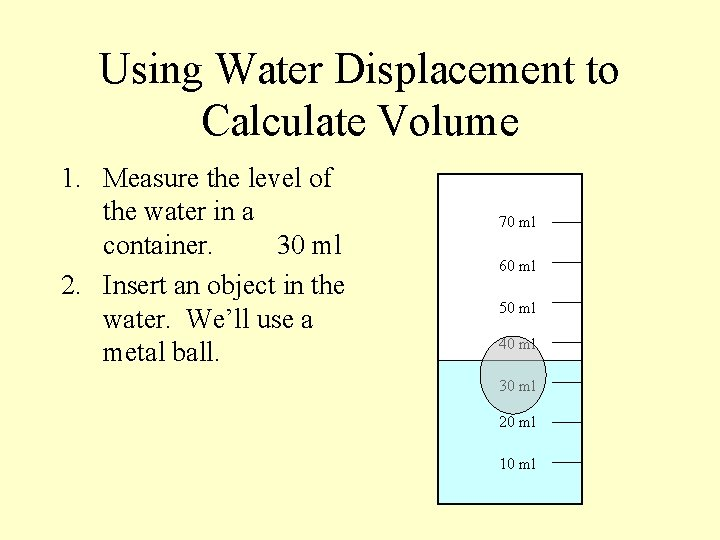Using Water Displacement to Calculate Volume 1. Measure the level of the water in a container. 30 ml 2. Insert an object in the water. We’ll use a metal ball. 70 ml 60 ml 50 ml 40 ml 30 ml 20 ml 10 ml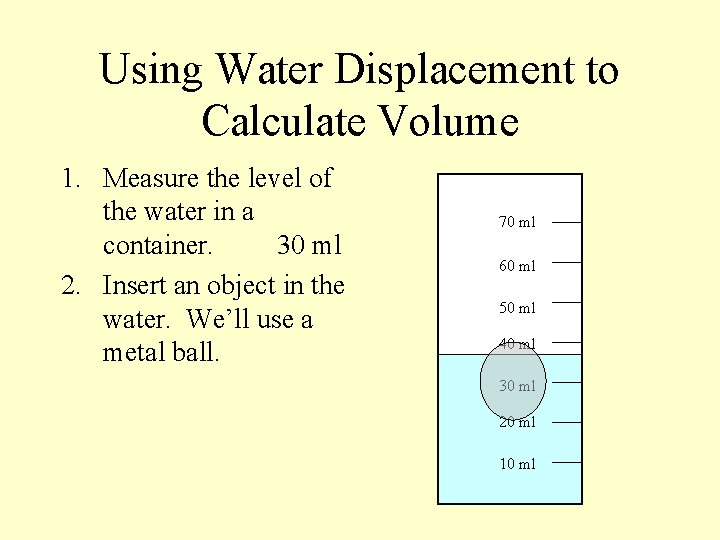Using Water Displacement to Calculate Volume 1. Measure the level of the water in a container. 30 ml 2. Insert an object in the water. We’ll use a metal ball. 70 ml 60 ml 50 ml 40 ml 30 ml 20 ml 10 mlUsing Water Displacement to Calculate Volume 1. Measure the level of the water in a container. 30 ml 2. Insert an object in the water. We’ll use a metal ball. 70 ml 60 ml 50 ml 40 ml 30 ml 20 ml 10 ml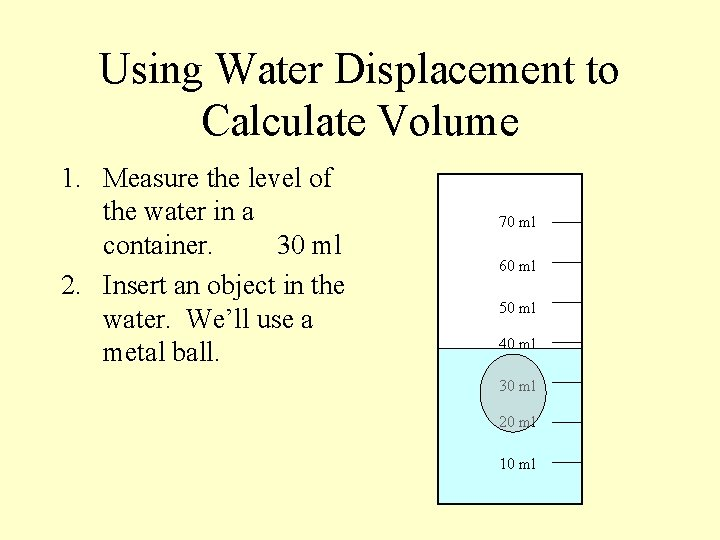Using Water Displacement to Calculate Volume 1. Measure the level of the water in a container. 30 ml 2. Insert an object in the water. We’ll use a metal ball. 70 ml 60 ml 50 ml 40 ml 30 ml 20 ml 10 mlUsing Water Displacement to Calculate Volume 1. Measure the level of the water in a container. 30 ml 2. Insert an object in the water. We’ll use a metal ball. 70 ml 60 ml 50 ml 40 ml 30 ml 20 ml 10 ml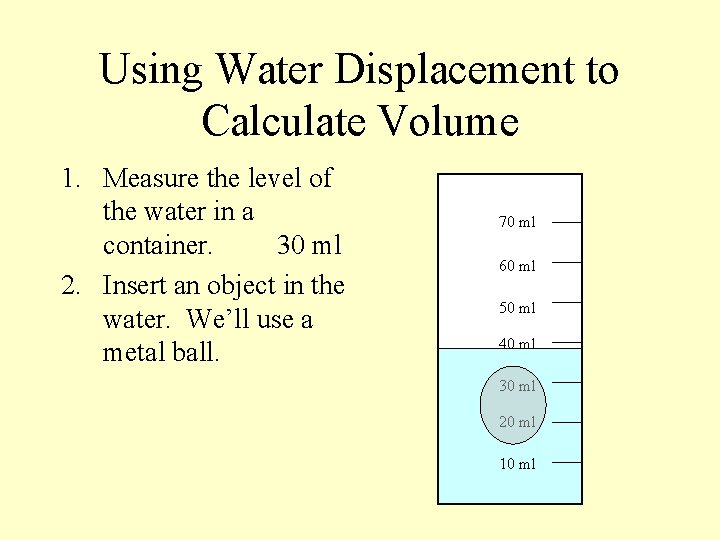Using Water Displacement to Calculate Volume 1. Measure the level of the water in a container. 30 ml 2. Insert an object in the water. We’ll use a metal ball. 70 ml 60 ml 50 ml 40 ml 30 ml 20 ml 10 mlUsing Water Displacement to Calculate Volume 1. Measure the level of the water in a container. 30 ml 2. Insert an object in the water. We’ll use a metal ball. 70 ml 60 ml 50 ml 40 ml 30 ml 20 ml 10 ml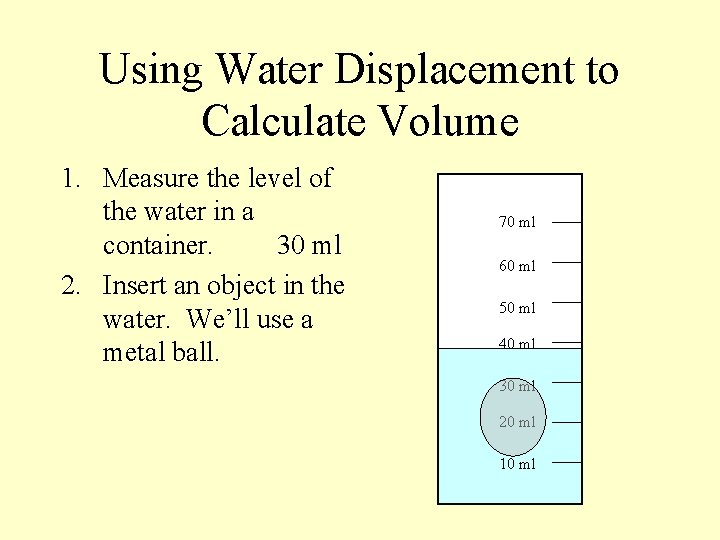Using Water Displacement to Calculate Volume 1. Measure the level of the water in a container. 30 ml 2. Insert an object in the water. We’ll use a metal ball. 70 ml 60 ml 50 ml 40 ml 30 ml 20 ml 10 mlUsing Water Displacement to Calculate Volume 1. Measure the level of the water in a container. 30 ml 2. Insert an object in the water. We’ll use a metal ball. 70 ml 60 ml 50 ml 40 ml 30 ml 20 ml 10 mlUsing Water Displacement to Calculate Volume 1. Measure the level of the water in a container. 30 ml 2. Insert an object in the water. We’ll use a metal ball. 70 ml 60 ml 50 ml 40 ml 30 ml 20 ml 10 ml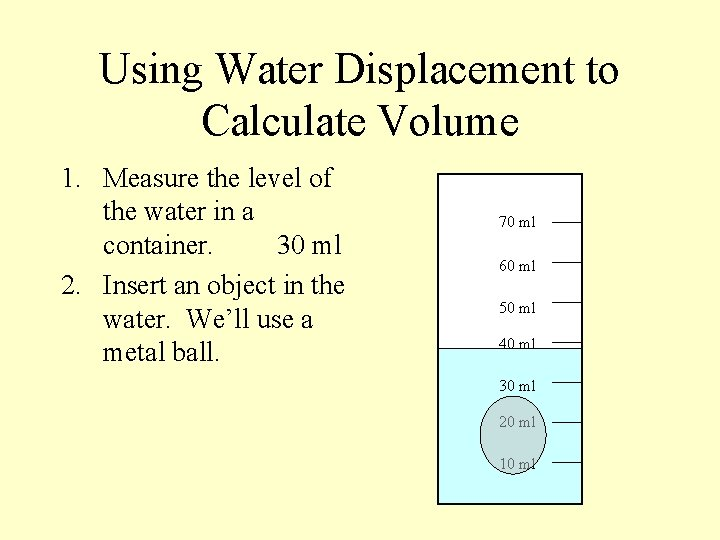Using Water Displacement to Calculate Volume 1. Measure the level of the water in a container. 30 ml 2. Insert an object in the water. We’ll use a metal ball. 70 ml 60 ml 50 ml 40 ml 30 ml 20 ml 10 mlUsing Water Displacement to Calculate Volume 1. Measure the level of the water in a container. 30 ml 2. Insert an object in the water. We’ll use a metal ball. 70 ml 60 ml 50 ml 40 ml 30 ml 20 ml 10 ml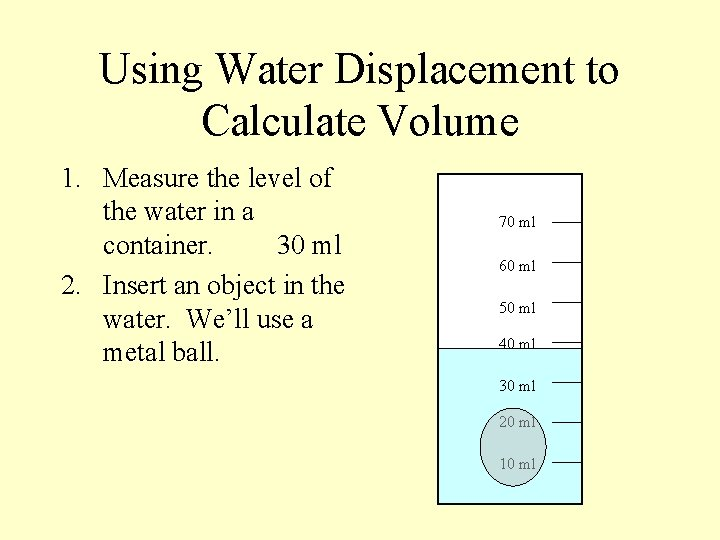Using Water Displacement to Calculate Volume 1. Measure the level of the water in a container. 30 ml 2. Insert an object in the water. We’ll use a metal ball. 70 ml 60 ml 50 ml 40 ml 30 ml 20 ml 10 mlUsing Water Displacement to Calculate Volume 1. Measure the level of the water in a container. 30 ml 2. Insert an object in the water. We’ll use a metal ball. 70 ml 60 ml 50 ml 40 ml 30 ml 20 ml 10 mlUsing Water Displacement to Calculate Volume 1. Measure the level of the water in a container. 30 ml 2. Insert an object in the water. We’ll use a metal ball. 70 ml 60 ml 50 ml 40 ml 30 ml 20 ml 10 ml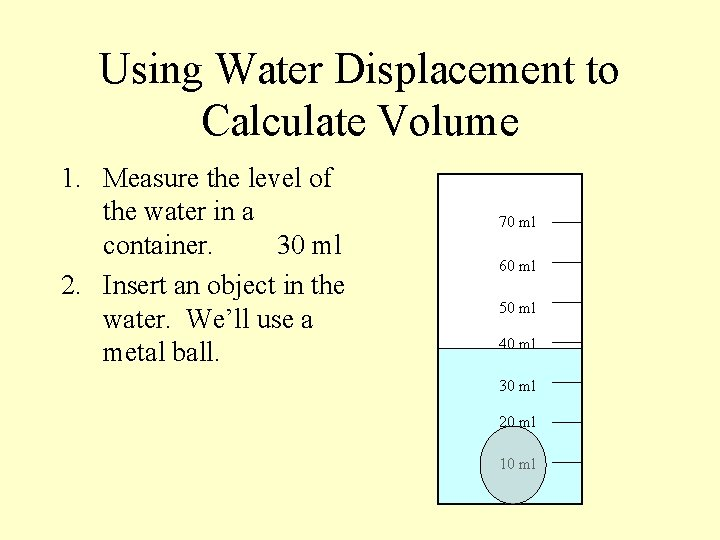Using Water Displacement to Calculate Volume 1. Measure the level of the water in a container. 30 ml 2. Insert an object in the water. We’ll use a metal ball. 70 ml 60 ml 50 ml 40 ml 30 ml 20 ml 10 mlUsing Water Displacement to Calculate Volume 1. Measure the level of the water in a container. 30 ml 2. Insert an object in the water. We’ll use a metal ball. 3. Measure the level after the ball has displaced some of the water. 70 ml 60 ml 50 ml 40 ml 30 ml 20 ml 10 ml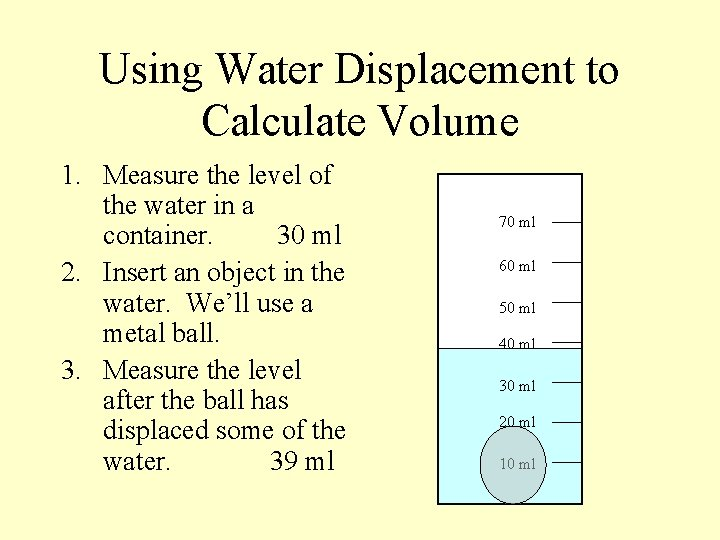Using Water Displacement to Calculate Volume 1. Measure the level of the water in a container. 30 ml 2. Insert an object in the water. We’ll use a metal ball. 3. Measure the level after the ball has displaced some of the water. 39 ml 70 ml 60 ml 50 ml 40 ml 30 ml 20 ml 10 mlUsing Water Displacement to Calculate Volume 1. 2. 3. 4. Measure the level of the water in a container. 30 ml Insert an object in the water. We’ll use a metal ball. Measure the level after the ball has displaced some of the water. 39 ml Finally, find the difference between the water level before displacement and after displacement. 39 minus 30 = 9 ml 70 ml 60 ml 50 ml 40 ml 30 ml 20 ml 10 ml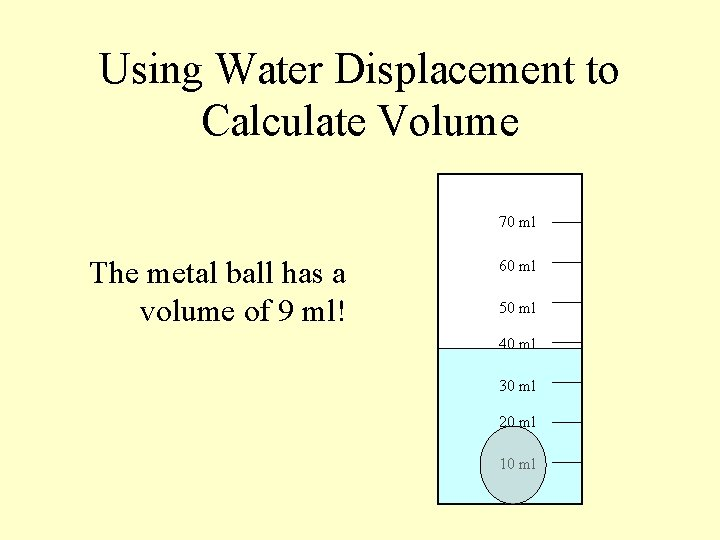Using Water Displacement to Calculate Volume 70 ml The metal ball has a volume of 9 ml! 60 ml 50 ml 40 ml 30 ml 20 ml 10 mlDensity • Density refers to “how crowded” something is. The amount of matter within a certain volume. • Density can be measured in grams per milliliter (g/ml). • Solids are the densest phase of matter. Gases are the least dense.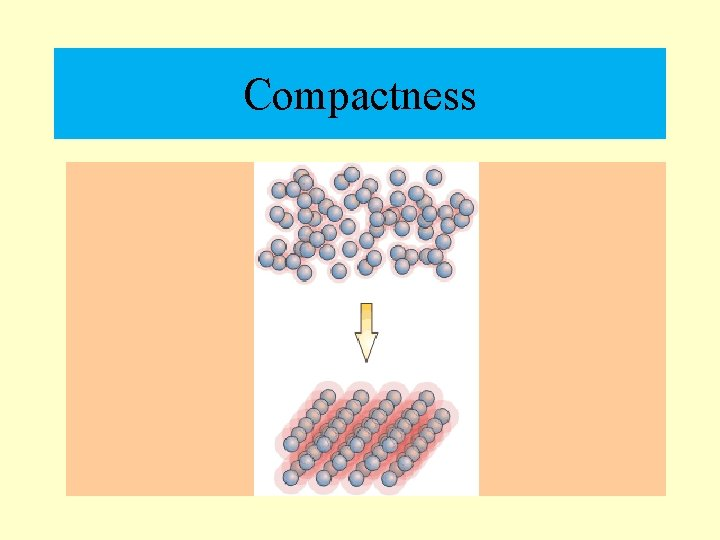Compactness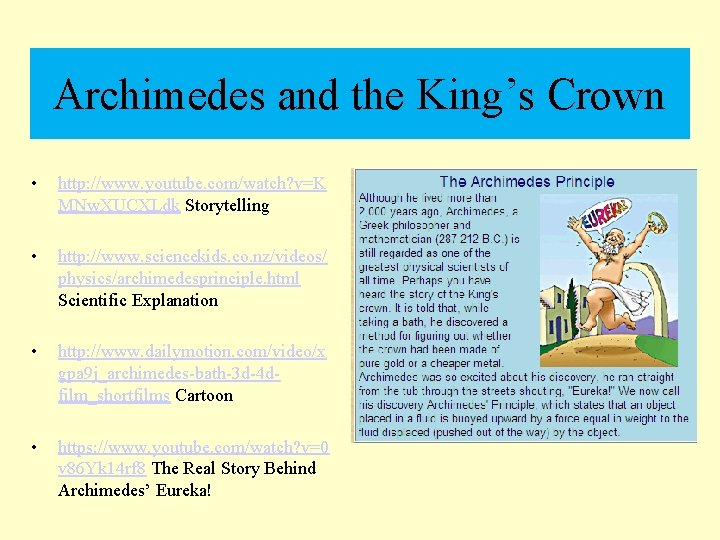Archimedes and the King’s Crown • http: //www. youtube. com/watch? v=K MNw. XUCXLdk Storytelling • http: //www. sciencekids. co. nz/videos/ physics/archimedesprinciple. html Scientific Explanation • http: //www. dailymotion. com/video/x gpa 9 j_archimedes-bath-3 d-4 dfilm_shortfilms Cartoon • https: //www. youtube. com/watch? v=0 v 86 Yk 14 rf 8 The Real Story Behind Archimedes’ Eureka!How to Calculate Density n Regular Shaped Solids – measure mass, then determine the volume by using a the formula Lx. Wx. H. EX: cubes, cylinders, spheres, cones, etc.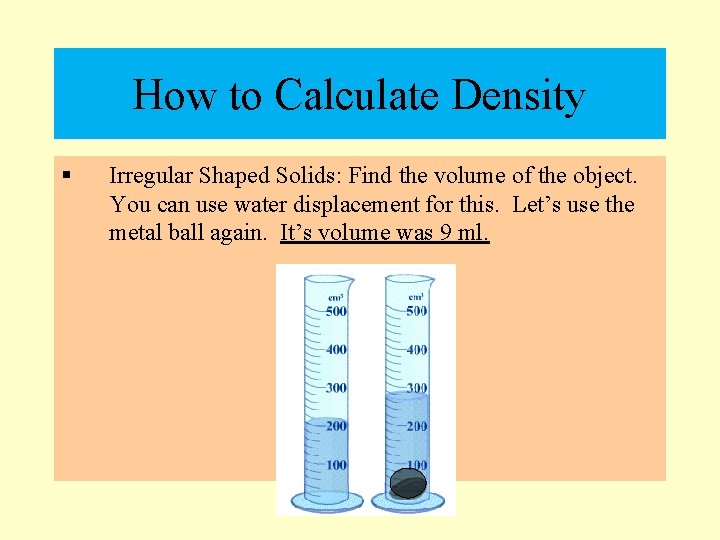How to Calculate Density § Irregular Shaped Solids: Find the volume of the object. You can use water displacement for this. Let’s use the metal ball again. It’s volume was 9 ml.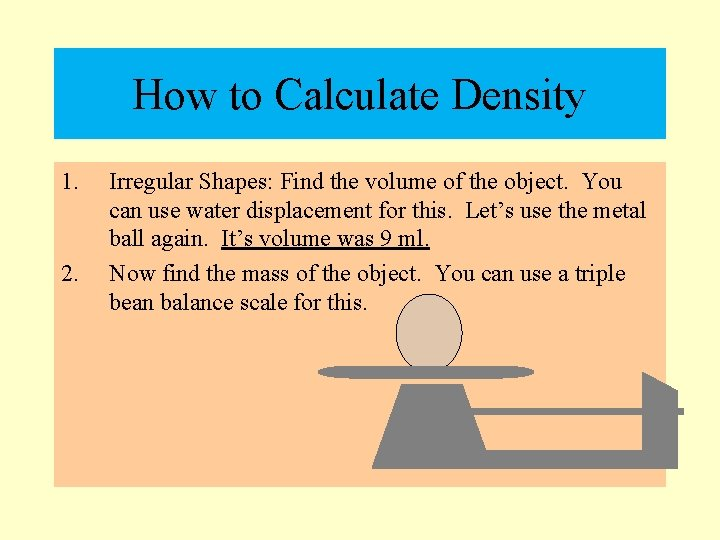How to Calculate Density 1. 2. Irregular Shapes: Find the volume of the object. You can use water displacement for this. Let’s use the metal ball again. It’s volume was 9 ml. Now find the mass of the object. You can use a triple bean balance scale for this.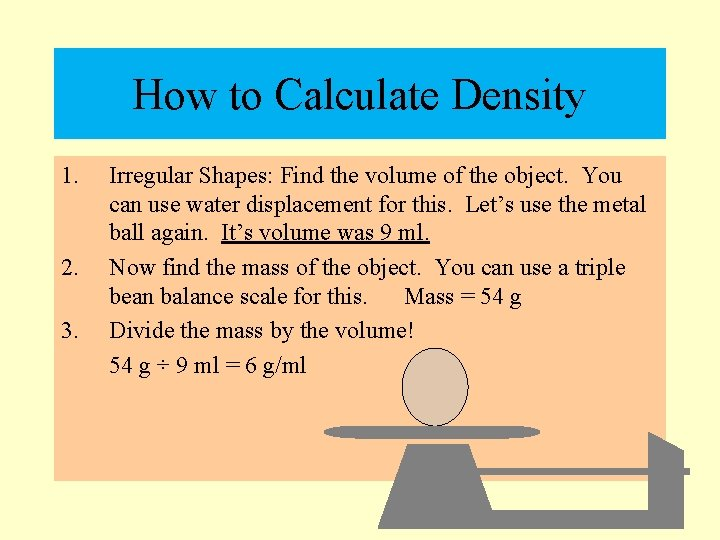How to Calculate Density 1. 2. 3. Irregular Shapes: Find the volume of the object. You can use water displacement for this. Let’s use the metal ball again. It’s volume was 9 ml. Now find the mass of the object. You can use a triple bean balance scale for this. Mass = 54 g Divide the mass by the volume! 54 g ÷ 9 ml = 6 g/ml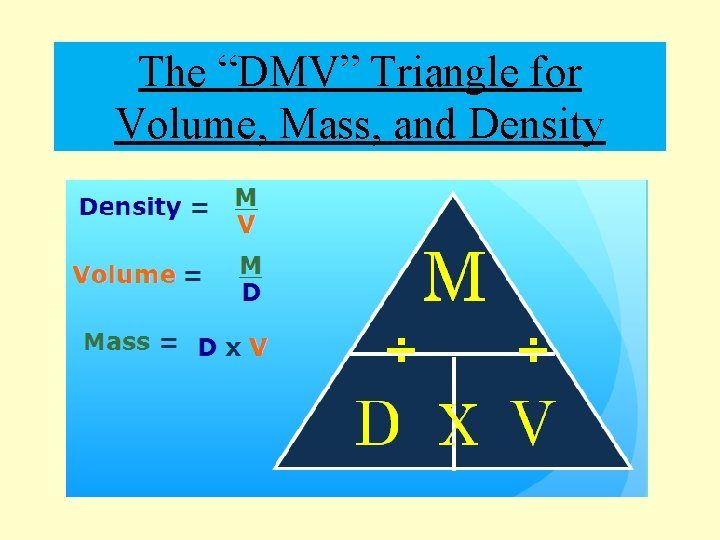The “DMV” Triangle for Volume, Mass, and DensityMore About Density. . . • Pure water has a density of 1 g/ml. • If any material is denser than the fluid that surrounds it, it will have negative buoyancy. It will sink! • If any material is less dense than the fluid that surrounds it, it will have positive buoyancy. It will float!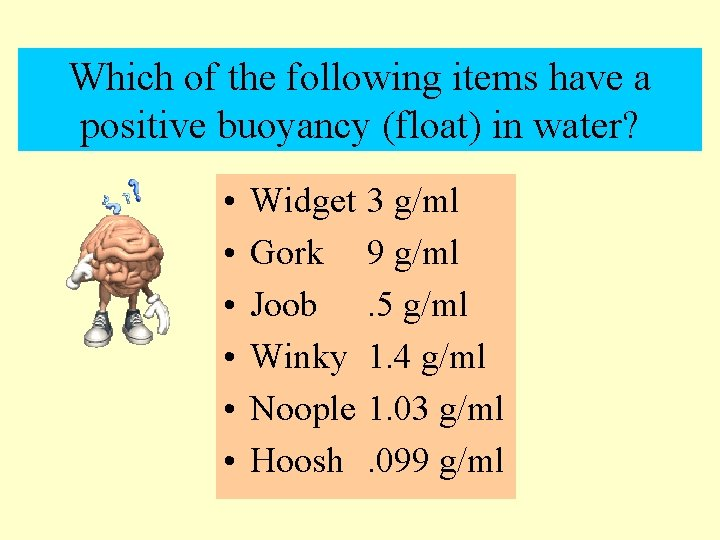Which of the following items have a positive buoyancy (float) in water? • • • Widget Gork Joob Winky Noople Hoosh 3 g/ml 9 g/ml. 5 g/ml 1. 4 g/ml 1. 03 g/ml. 099 g/mlWhich of the following items have a positive buoyancy (float) in water? • • • Widget Gork Joob Winky Noople Hoosh 3 g/ml 9 g/ml. 5 g/ml 1. 4 g/ml 1. 03 g/ml. 099 g/ml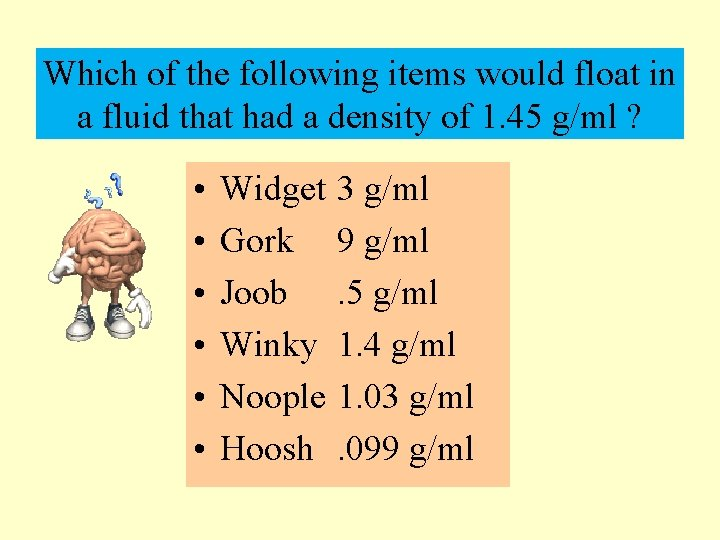Which of the following items would float in a fluid that had a density of 1. 45 g/ml ? • • • Widget Gork Joob Winky Noople Hoosh 3 g/ml 9 g/ml. 5 g/ml 1. 4 g/ml 1. 03 g/ml. 099 g/mlWhich of the following items would float in a fluid that had a density of 1. 45 g/ml ? • • • Widget Gork Joob Winky Noople Hoosh 3 g/ml 9 g/ml. 5 g/ml 1. 4 g/ml 1. 03 g/ml. 099 g/ml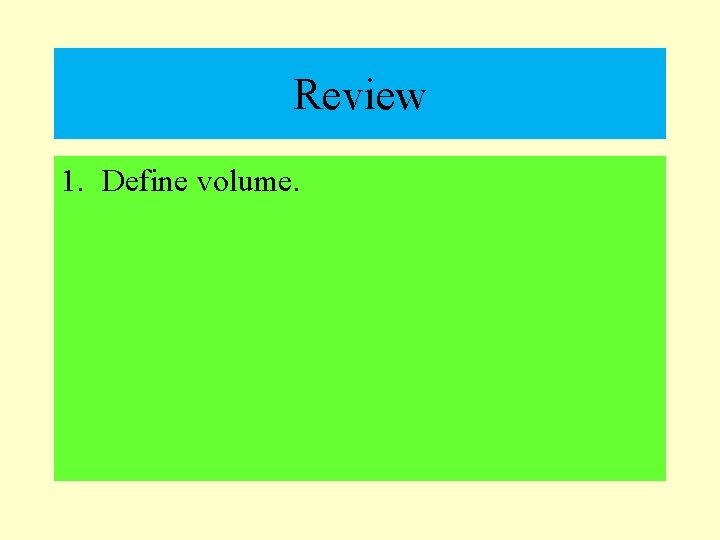Review 1. Define volume.Review 1. Define volume. 2. Identify the liquid that has a volume of 1 g/ml?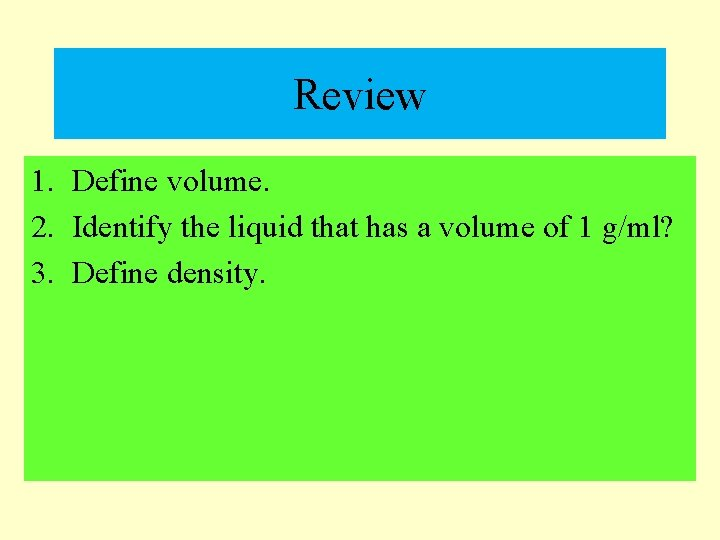Review 1. Define volume. 2. Identify the liquid that has a volume of 1 g/ml? 3. Define density.Review 1. 2. 3. 4. Define volume. Identify the liquid that has a volume of 1 g/ml? Define density. Explain water displacement.Review 1. 2. 3. 4. 5. Define volume. Identify the liquid that has a volume of 1 g/ml? Define density. Explain water displacement. Identify the density of a substance that would sink when placed in water.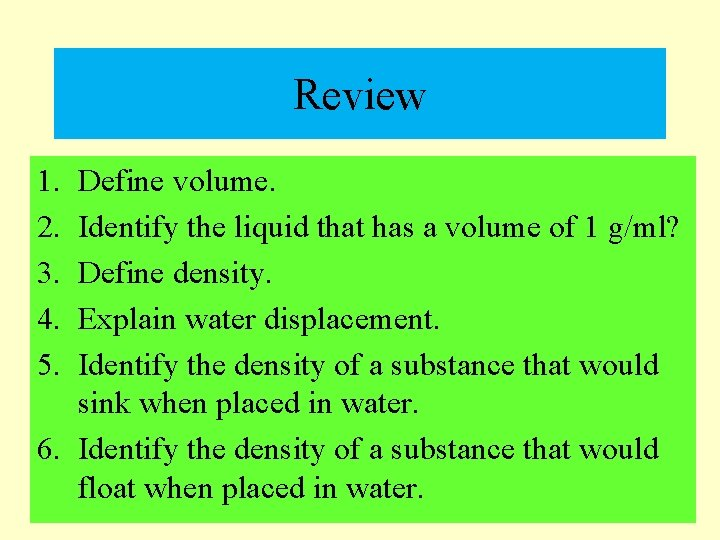Review 1. 2. 3. 4. 5. Define volume. Identify the liquid that has a volume of 1 g/ml? Define density. Explain water displacement. Identify the density of a substance that would sink when placed in water. 6. Identify the density of a substance that would float when placed in water.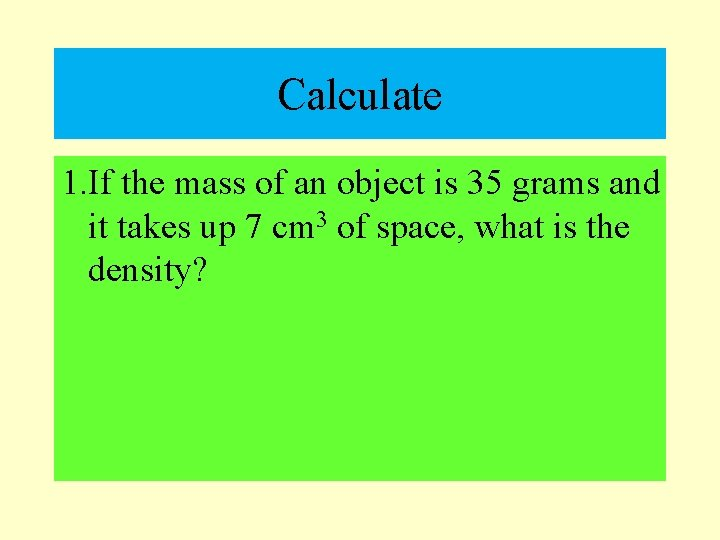Calculate 1. If the mass of an object is 35 grams and it takes up 7 cm 3 of space, what is the density?Enrichment: How does density affect life?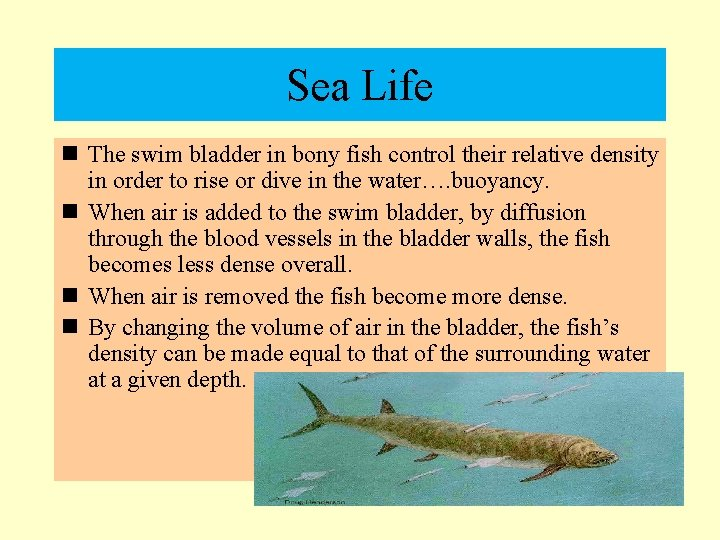Sea Life n The swim bladder in bony fish control their relative density in order to rise or dive in the water…. buoyancy. n When air is added to the swim bladder, by diffusion through the blood vessels in the bladder walls, the fish becomes less dense overall. n When air is removed the fish become more dense. n By changing the volume of air in the bladder, the fish’s density can be made equal to that of the surrounding water at a given depth.Boats If the boat floats then it is less dense than the water. If it sinks, then it's denser than the water. Fresh water would generally be less dense than salt water. Swimming also deals with density. Basically you need enough oxygen in your body to make yourself less dense than the water that way you can float.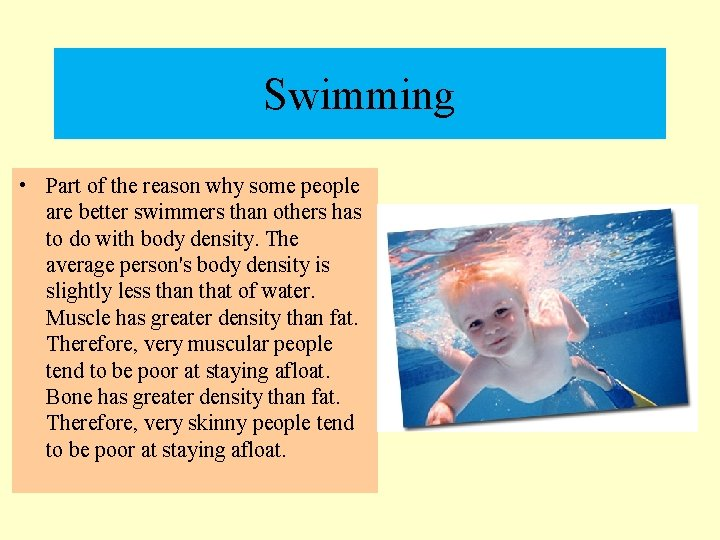Swimming • Part of the reason why some people are better swimmers than others has to do with body density. The average person's body density is slightly less than that of water. Muscle has greater density than fat. Therefore, very muscular people tend to be poor at staying afloat. Bone has greater density than fat. Therefore, very skinny people tend to be poor at staying afloat.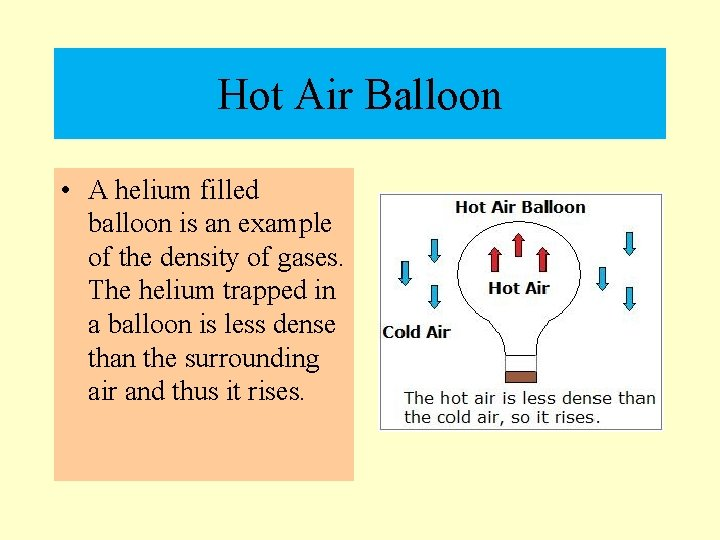Hot Air Balloon • A helium filled balloon is an example of the density of gases. The helium trapped in a balloon is less dense than the surrounding air and thus it rises.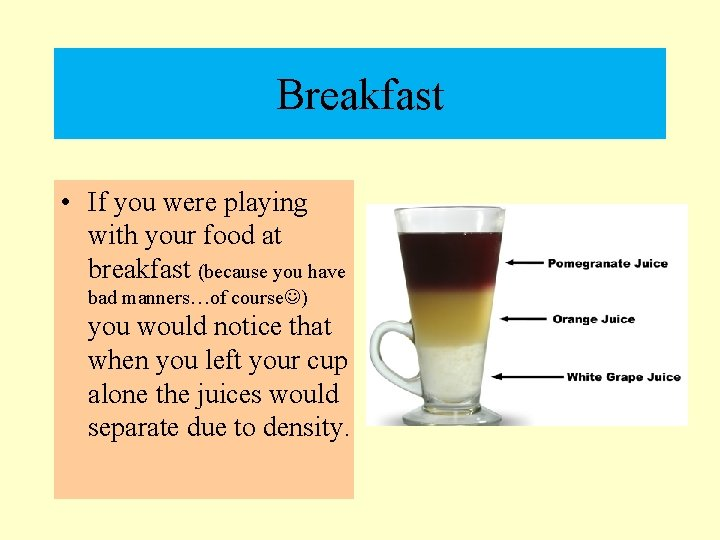Breakfast • If you were playing with your food at breakfast (because you have bad manners…of course ) you would notice that when you left your cup alone the juices would separate due to density.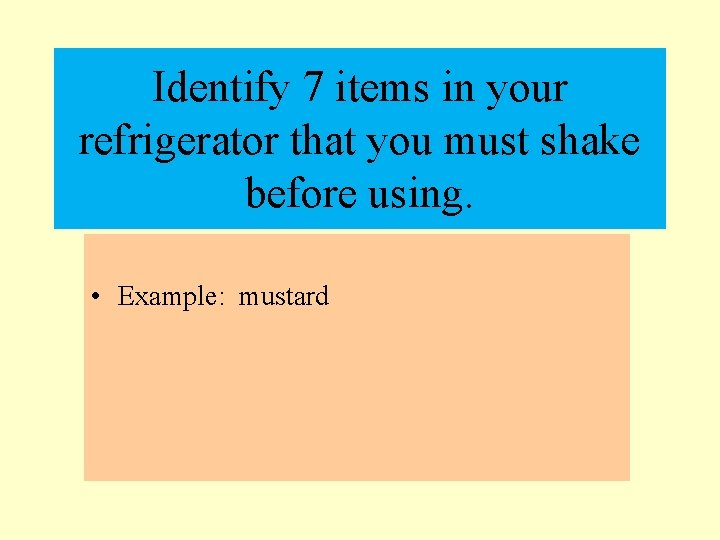Identify 7 items in your refrigerator that you must shake before using. • Example: mustard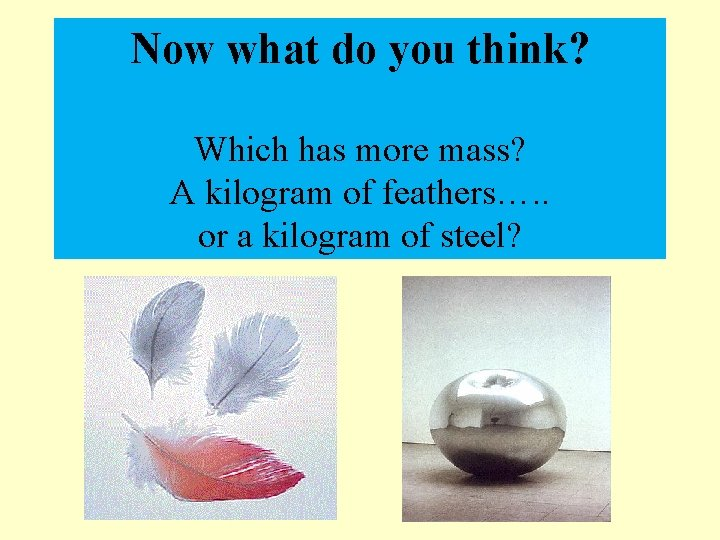Now what do you think? Which has more mass? A kilogram of feathers…. . or a kilogram of steel?